# bf 的博客# bf 的博客

### 浅谈红黑树

posted on 2020-02-28 09:49:29 | under 未分类 |

## 浅谈红黑树

### 0 说明

$rnk$ 排名， $kth$ 排名为第 $k$ 的数， $suf$ 后缀， $pre$ 前驱， $ins$ 插入， $del$ 删除， $ins\_fix$ 插入修正（双红修正）， $del\_fix$ 删除修正（双黑修正）。

### 1 性质

• $1$ 一棵二叉搜索树，所有节点均为红色或黑色。
• 相信这一条性质无需多言了。
• $2$ 根节点为黑色。
• 根节点为黑色，会给我们后面的修正带来很大的便利。
• 另外，红黑树的黑高度变化全部在根节点产生，黑高度的定义在后文会有提及。
• $3$ 所有叶节点为黑色。
• 一般来说，我们会认为增加一层，也就是在叶节点的下放加一层 $0$ 节点，并令 $0$ 为黑色。
• 如果使用指针，那么就是叶节点的指针指向 $null$ 。
• $4$ 所有红节点的子节点均为黑节点。
• 这是一条保证红黑树复杂度的性质，使得每一个点到根节点的距离不超过黑高度的 $2$ 倍。
• $5$ 所有的叶节点到根节点的路径上的黑节点数量相同。
• 这一个数量也称就是黑高度。
• 由于存在这一条性质，我们可以保证每一个点到根节点路径上的黑点不超过 $\log n+1$ 。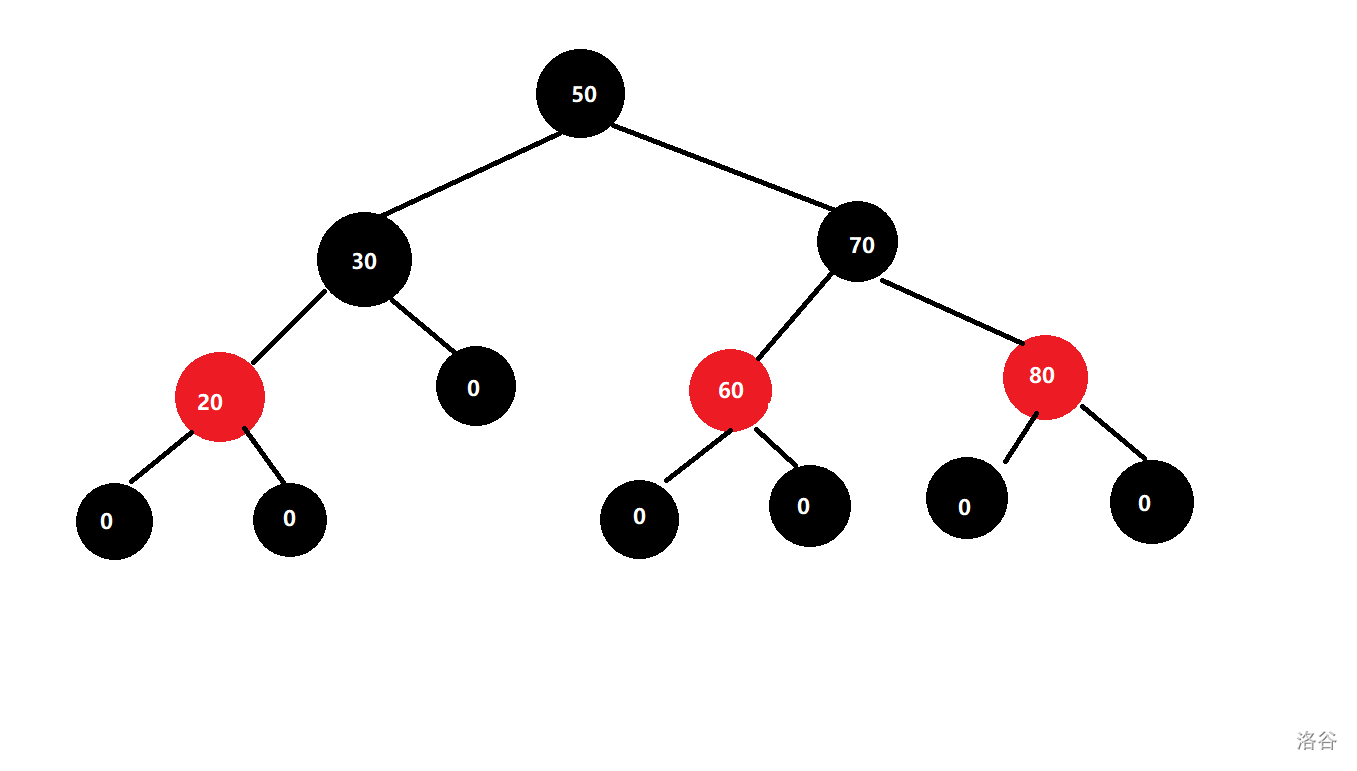### 2 查询操作

int kth(int k) {
int tmp;
int o=rt;
for (;o;) {
tmp=t[t[o].ch].sz;
if (tmp+1<=k&&k<=tmp+t[o].cnt)
break;
else{
if (k<=tmp)
o=t[o].ch;
else{
k-=tmp+t[o].cnt;
o=t[o].ch;
}
}
}
return t[o].v;
}
int rnk(int v) {
ins(v);
int tmp=0,sum=0;
int o=rt;
for (;o;) {
tmp=t[t[o].ch].sz;
if(v==t[o].v)
break;
else{
if(v<t[o].v)
o=t[o].ch;
else{
sum+=tmp+t[o].cnt,
o=t[o].ch;
}
}
}
del(v);
return sum+tmp+1;
}
int suf(int v) {
int res=inf;
int o=rt;
for(;o;){
if(t[o].v>v){
res=t[o].v,
o=t[o].ch;
}
else
o=t[o].ch;
}
return res;
}
int pre(int v) {
int res=-inf;
int o=rt;
for(;o;){
if(t[o].v<v){
res=t[o].v,o=t[o].ch;
}
else
o=t[o].ch;
}
return res;
}

### 3 旋转操作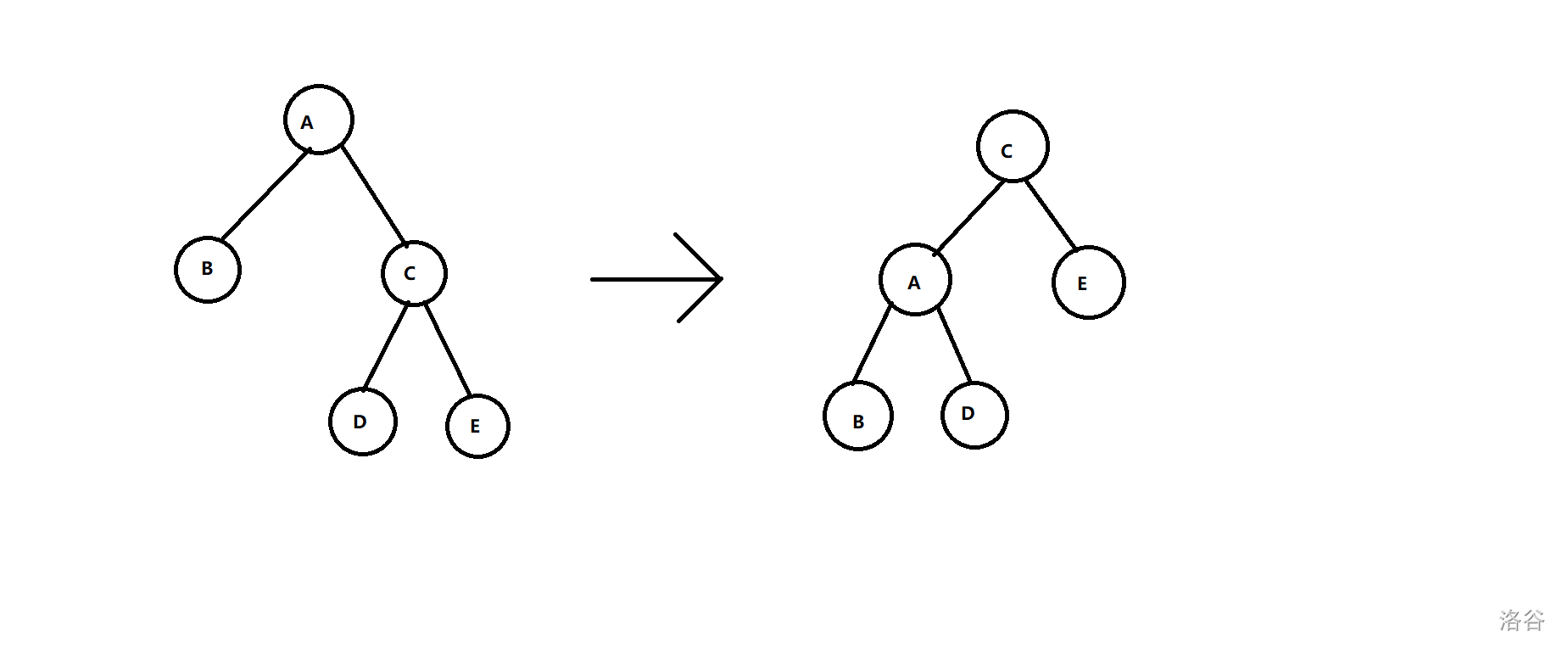void pushup(int o){
t[o].sz=t[t[o].ch].sz+t[t[o].ch].sz+t[o].cnt;
}
bool get(int o){
return t[t[o].fa].ch==o;
}
void rotate(int o,bool c){
int s1=t[o].ch[!c];
t[o].ch[!c]=t[s1].ch[c];
if (t[s1].ch[c])
t[t[s1].ch[c]].fa=o;
t[s1].fa=t[o].fa;
if(!t[o].fa)
rt=s1;
else
t[t[o].fa].ch[get(o)]=s1;
t[s1].ch[c]=o;
t[o].fa=s1;
pushup(o);
pushup(s1);
}

### 4 插入操作

• $1$ 祖父节点的另一个子节点为黑色。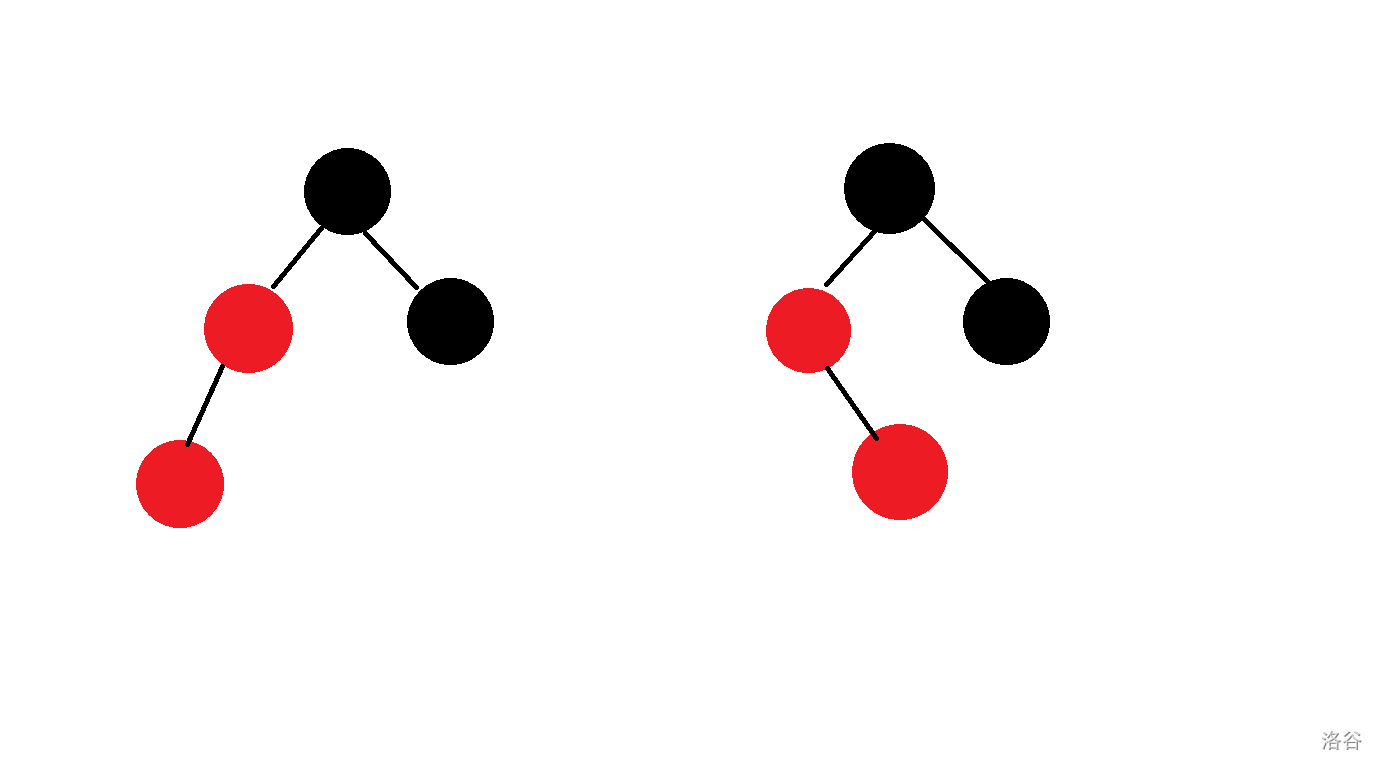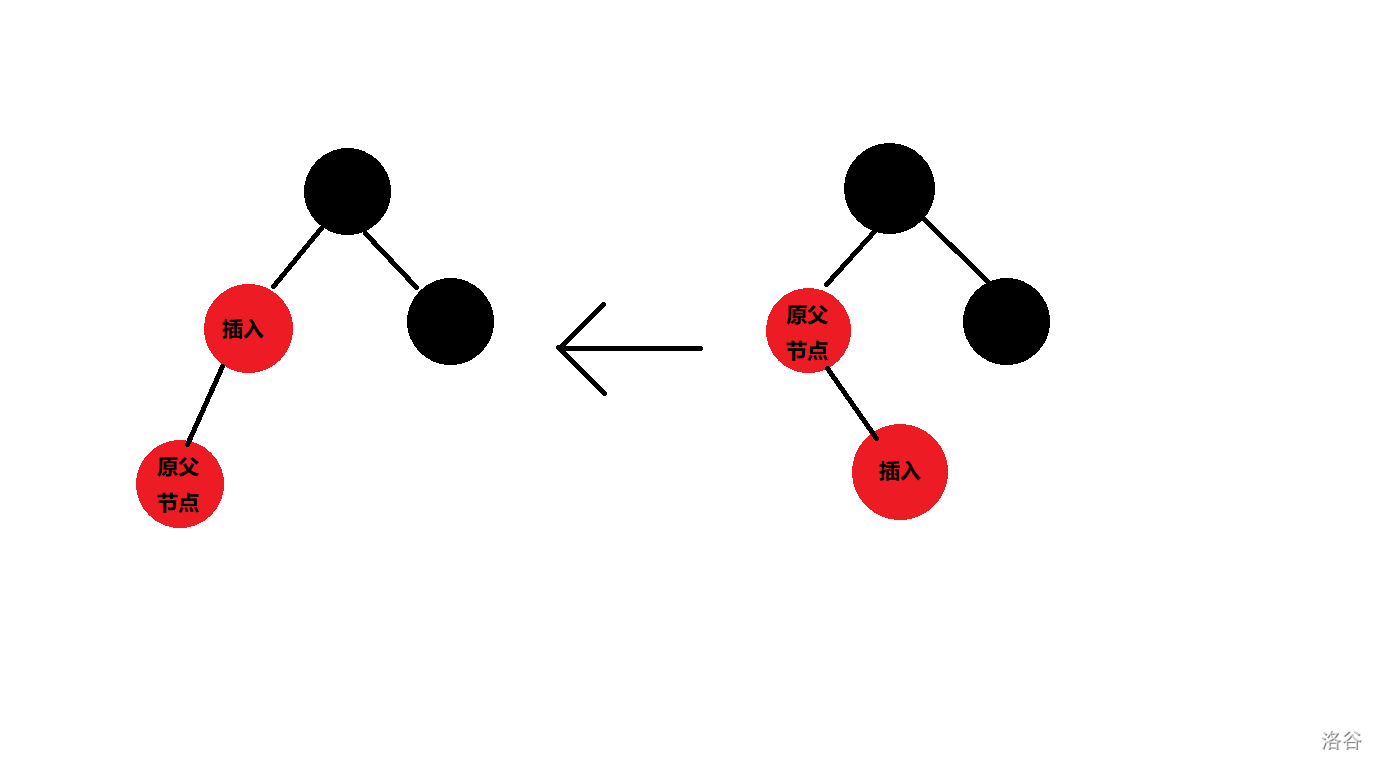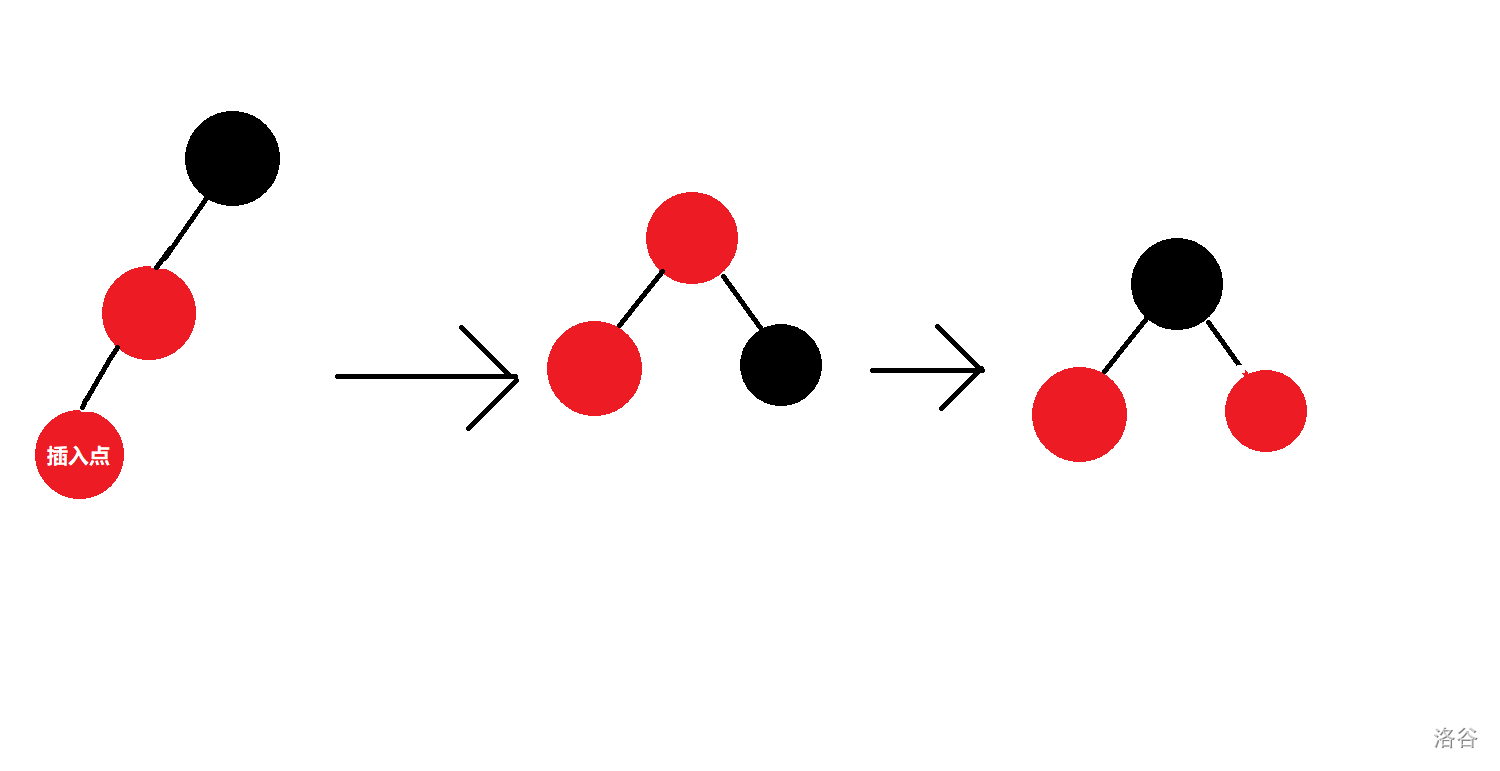• $2$ 祖父节点的另一子节点也是红节点。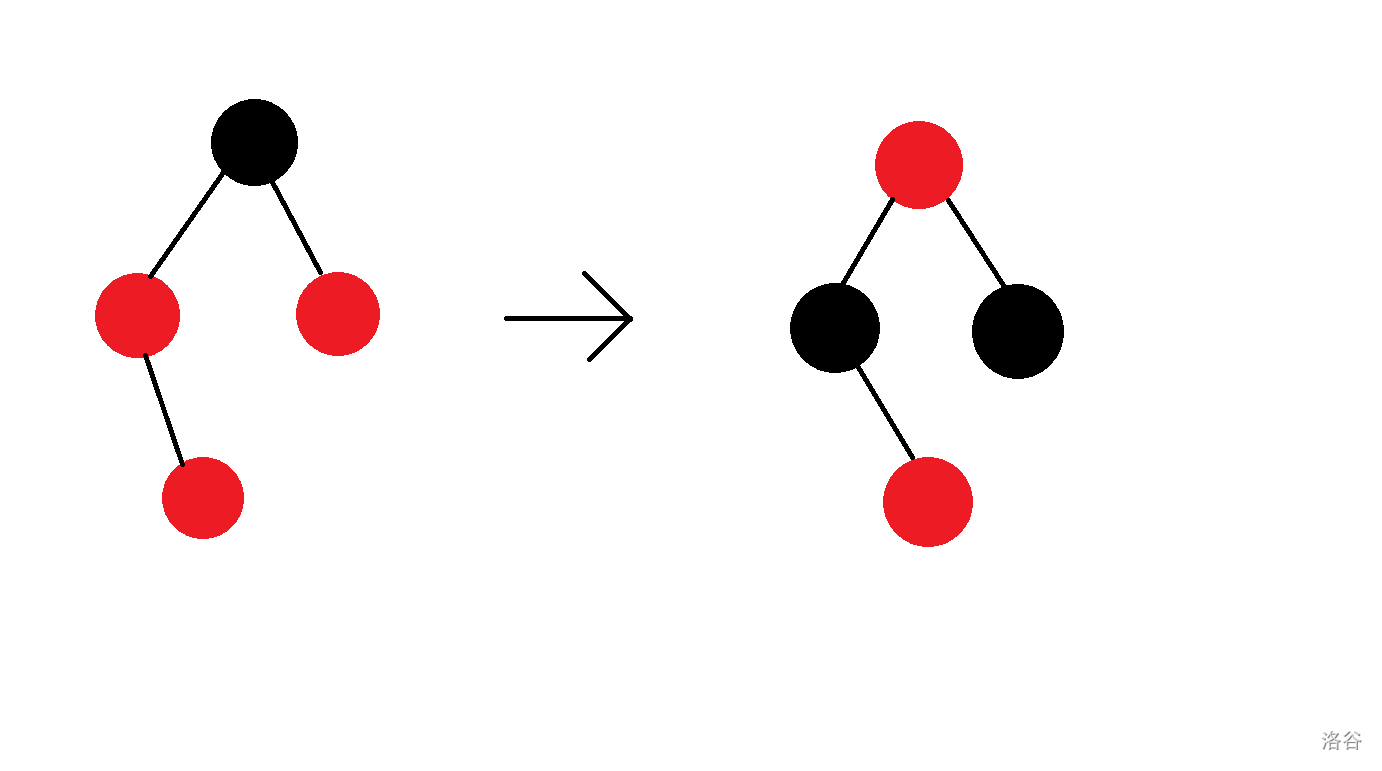int newnode(int v){
int o=top?st[top--]:++tot;
t[o]=(rbt){v,1,1,1,0,0,0};
return o;
}
void ins_fix(int o) {
while(t[t[o].fa].col){
int f=t[o].fa,gf=t[f].fa;
bool wh=!get(f);
int ul=t[gf].ch[wh];
if(t[ul].col) {
t[f].col=t[ul].col=0;
t[gf].col=1;
o=gf;
}
else{
if(o==t[f].ch[wh])
rotate(o=f,!wh);
else{
t[gf].col=1;
t[f].col=0;
rotate(gf,wh);
}
}
}
t[rt].col=0;
}
void ins(int v) {
int o=rt,f=0;
while(o){
t[o].sz++,f=o;
if(v==t[o].v){
t[o].cnt++;
return;
}
o=t[o].ch[v>t[o].v];
}
o=newnode(v);
if(f) t[f].ch[v>t[f].v]=o;
else rt=o;
t[o].fa=f;
ins_fix(o);
}

### 5 删除操作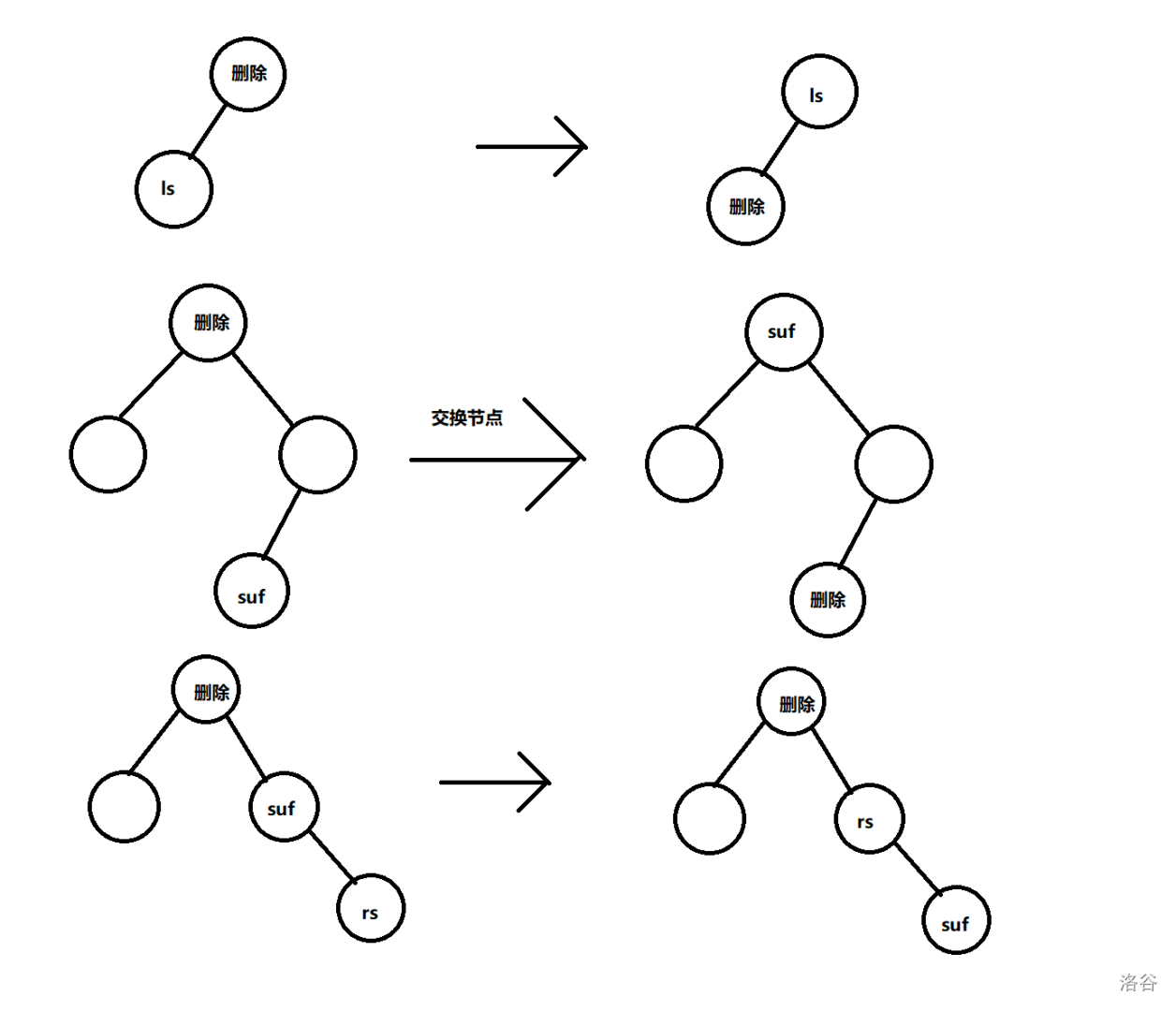• $1$ 父节点是黑色，其另一子节点是黑色，且这一节点的两个子节点至少有一个是红色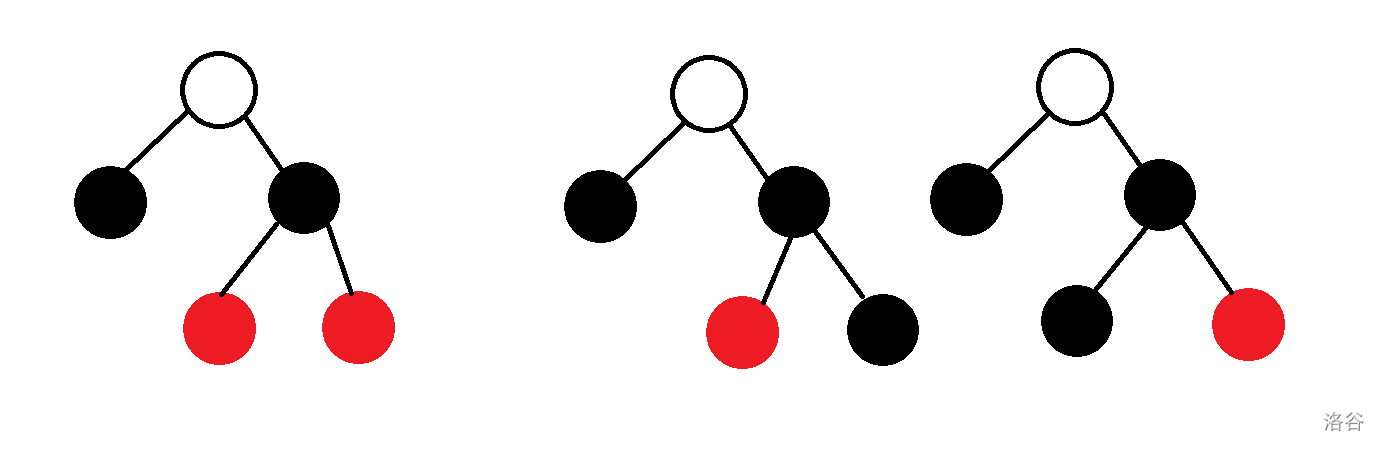（空节点代表颜色任意）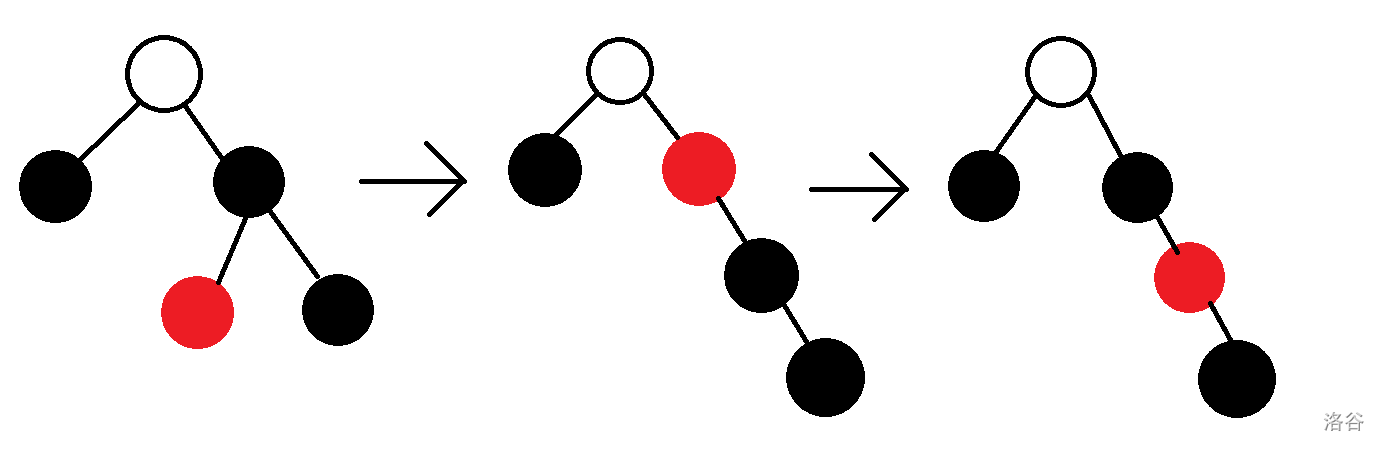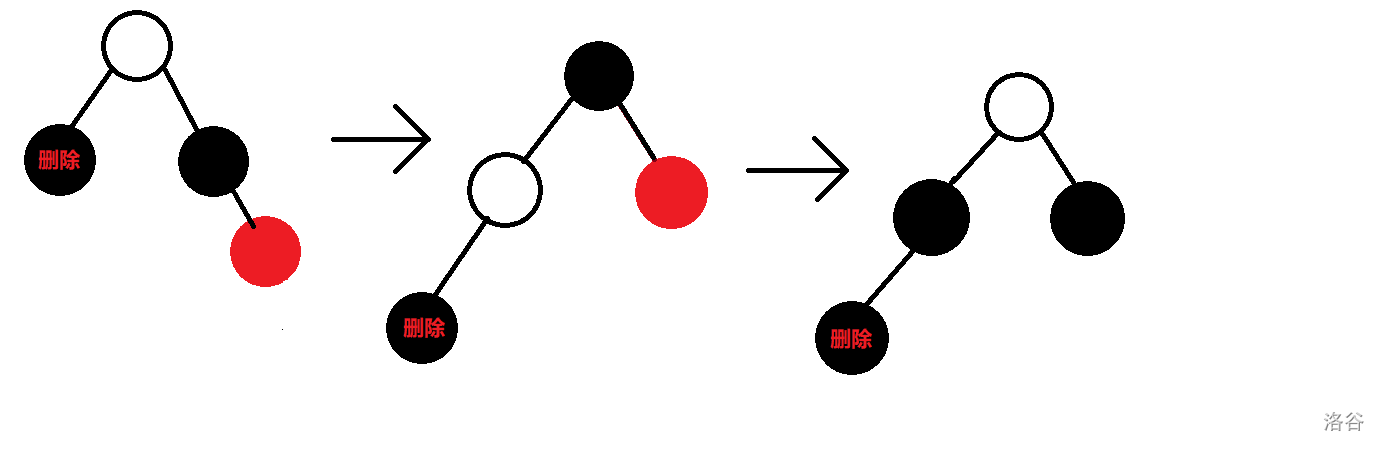• $2$ 祖父节点的另一子节点的两个儿子均为黑色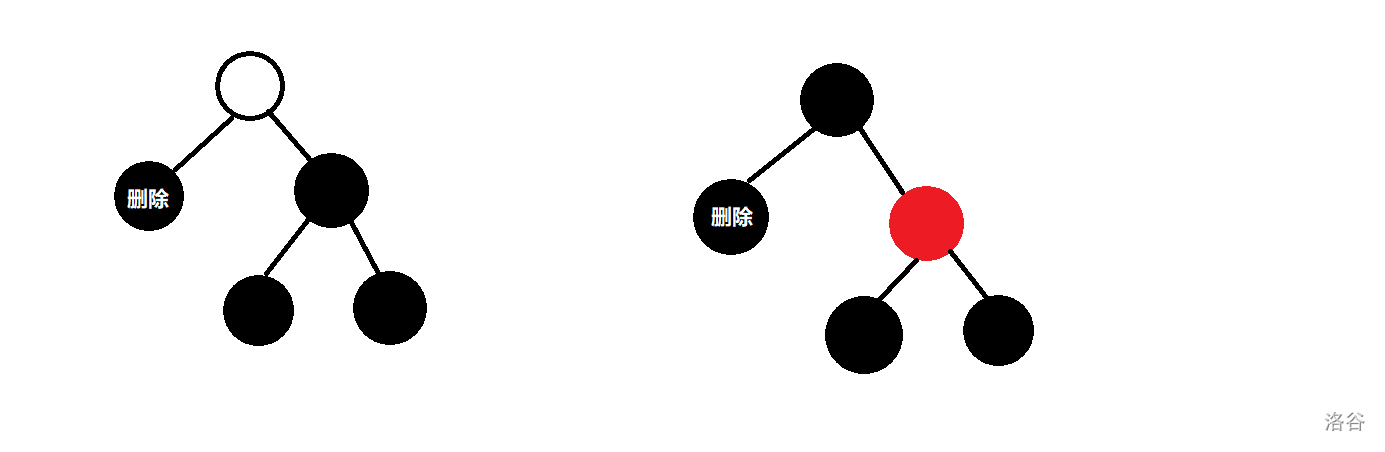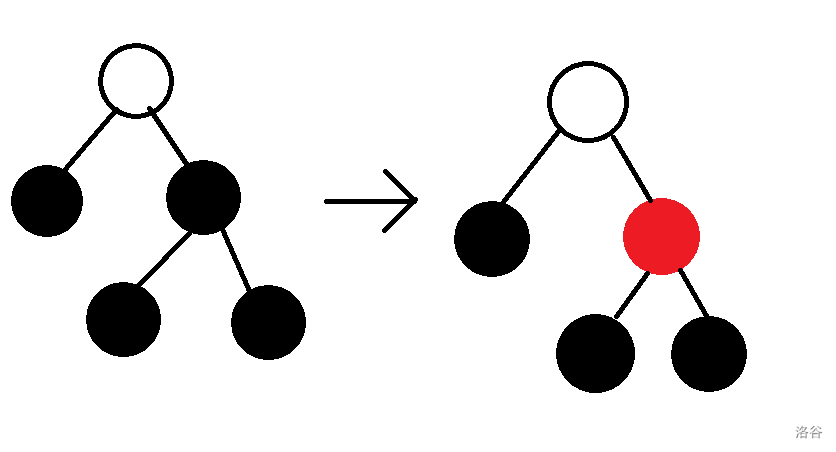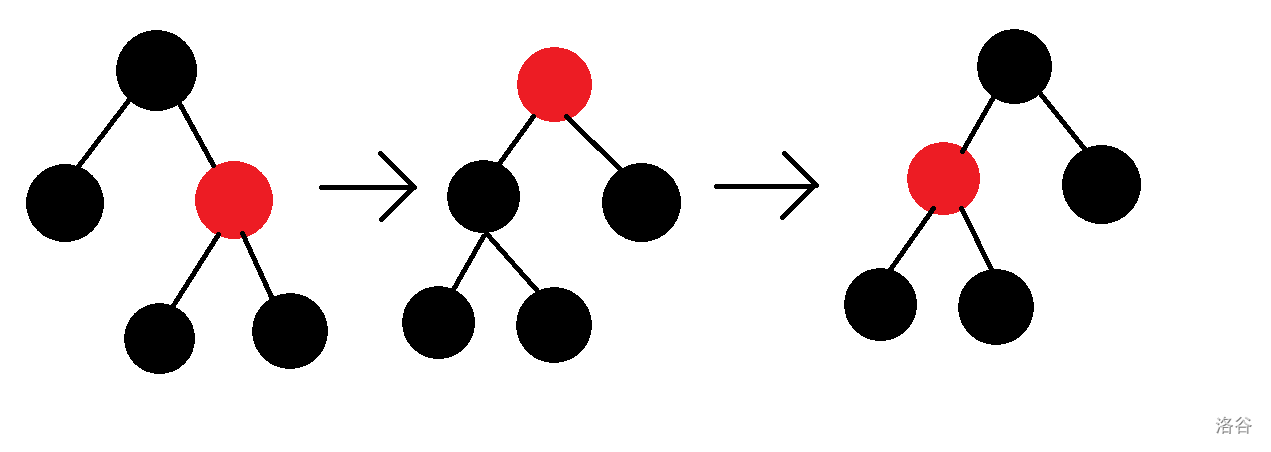void del_fix(int o) {
while(o!=rt&&!t[o].col) {
bool wh=!get(o);
int f=t[o].fa,ul=t[f].ch[wh];
if(t[ul].col){
t[ul].col=0;
t[f].col=1;
rotate(f,!wh);
ul=t[f].ch[wh];
}
else{
if(!t[t[ul].ch].col&&!t[t[ul].ch].col){
t[ul].col=1;
o=f;
}
else{
if(!t[t[ul].ch[wh]].col){
t[t[ul].ch[!wh]].col=0;
t[ul].col=1;
rotate(ul,wh);
ul=t[f].ch[wh];
}
t[ul].col=t[f].col;
t[t[ul].ch[wh]].col=t[f].col=0;
rotate(f,!wh);
break;
}
}
}
t[o].col=0;
}
void del(int v) {
int o=rt;
while(o&&t[o].v!=v){
o=t[o].ch[t[o].v<v];
}
if(!o)return;
if(t[o].cnt>1) {
t[o].cnt--;
update(o);
return;
}
int d=o,g=0;
if(t[o].ch&&t[o].ch){
d=t[o].ch;
while(t[d].ch)
d=t[d].ch;
}
g=t[d].ch[!t[d].ch];
t[g].fa=t[d].fa;
if(!t[d].fa)
rt=g;
else t[t[d].fa].ch[get(d)]=g;
if(o!=d){
t[o].v=t[d].v;
t[o].cnt=t[d].cnt;
}
update(t[d].fa);
for(int i=t[d].fa;i&&t[d].cnt>1&&i!=o;i=t[i].fa){
t[i].sz-=t[d].cnt;
t[i].sz++;
}
if (!t[d].col) del_fix(g);
st[++top]=d;
}

### 6 总结

#include <bits/stdc++.h>
using namespace std;
register int x=0;
register bool f=0;
register char c=getchar();
while(c<'0'||c>'9'){
if(c=='-') f=1;
c=getchar();
}
while(c>='0'&&c<='9'){
x=(x<<3)+(x<<1)+c-48;
c=getchar();
}
return f?-x:x;
}
char cr;int tt;
inline void print(int x,char k='\n') {
if(!x) putchar('0');
if(x < 0) putchar('-'),x=-x;
while(x) cr[++tt]=x%10+'0',x/=10;
while(tt) putchar(cr[tt--]);
putchar(k);
}
const int inf=2147483647;
const int maxn=1100100;
struct rbt{
int v,sz,cnt;
bool col;
int fa,ch;
}t[maxn*4];
int st[maxn*4],top,rt,tot,n,m,ans,lastans;
void pushup(int o){
t[o].sz=t[t[o].ch].sz+t[t[o].ch].sz+t[o].cnt;
}
bool get(int o){
return t[t[o].fa].ch==o;
}
int newnode(int v){
int o=top?st[top--]:++tot;
t[o]=(rbt){v,1,1,1,0,0,0};
return o;
}
void rotate(int o,bool c){
int s1=t[o].ch[!c];
t[o].ch[!c]=t[s1].ch[c];
if (t[s1].ch[c])
t[t[s1].ch[c]].fa=o;
t[s1].fa=t[o].fa;
if(!t[o].fa)
rt=s1;
else
t[t[o].fa].ch[get(o)]=s1;
t[s1].ch[c]=o;
t[o].fa=s1;
pushup(o);
pushup(s1);
}
void ins_fix(int o) {
while(t[t[o].fa].col){
int f=t[o].fa,gf=t[f].fa;
bool wh=!get(f);
int ul=t[gf].ch[wh];
if(t[ul].col) {
t[f].col=t[ul].col=0;
t[gf].col=1;
o=gf;
}
else{
if(o==t[f].ch[wh])
rotate(o=f,!wh);
else{
t[gf].col=1;
t[f].col=0;
rotate(gf,wh);
}
}
}
t[rt].col=0;
}
void ins(int v) {
int o=rt,f=0;
while(o){
t[o].sz++,f=o;
if(v==t[o].v){
t[o].cnt++;
return;
}
o=t[o].ch[v>t[o].v];
}
o=newnode(v);
if(f) t[f].ch[v>t[f].v]=o;
else rt=o;
t[o].fa=f;
ins_fix(o);
}
int kth(int k) {
int tmp;
int o=rt;
for (;o;) {
tmp=t[t[o].ch].sz;
if (tmp+1<=k&&k<=tmp+t[o].cnt)
break;
else{
if (k<=tmp)
o=t[o].ch;
else{
k-=tmp+t[o].cnt;
o=t[o].ch;
}
}
}
return t[o].v;
}
void update(int o){
for(int i=o;i;i=t[i].fa){
t[i].sz--;
}
}
void del_fix(int o) {
while(o!=rt&&!t[o].col) {
bool wh=!get(o);
int f=t[o].fa,ul=t[f].ch[wh];
if(t[ul].col){
t[ul].col=0;
t[f].col=1;
rotate(f,!wh);
ul=t[f].ch[wh];
}
else{
if(!t[t[ul].ch].col&&!t[t[ul].ch].col){
t[ul].col=1;
o=f;
}
else{
if(!t[t[ul].ch[wh]].col){
t[t[ul].ch[!wh]].col=0;
t[ul].col=1;
rotate(ul,wh);
ul=t[f].ch[wh];
}
t[ul].col=t[f].col;
t[t[ul].ch[wh]].col=t[f].col=0;
rotate(f,!wh);
break;
}
}
}
t[o].col=0;
}
void del(int v) {
int o=rt;
while(o&&t[o].v!=v){
o=t[o].ch[t[o].v<v];
}
if(!o)return;
if(t[o].cnt>1) {
t[o].cnt--;
update(o);
return;
}
int d=o,g=0;
if(t[o].ch&&t[o].ch){
d=t[o].ch;
while(t[d].ch)
d=t[d].ch;
}
g=t[d].ch[!t[d].ch];
t[g].fa=t[d].fa;
if(!t[d].fa)
rt=g;
else t[t[d].fa].ch[get(d)]=g;
if(o!=d){
t[o].v=t[d].v;
t[o].cnt=t[d].cnt;
}
update(t[d].fa);
for(int i=t[d].fa;i&&t[d].cnt>1&&i!=o;i=t[i].fa){
t[i].sz-=t[d].cnt;
t[i].sz++;
}
if (!t[d].col) del_fix(g);
st[++top]=d;
}
int rnk(int v) {
ins(v);
int tmp=0,sum=0;
int o=rt;
for (;o;) {
tmp=t[t[o].ch].sz;
if(v==t[o].v)
break;
else{
if(v<t[o].v)
o=t[o].ch;
else{
sum+=tmp+t[o].cnt,
o=t[o].ch;
}
}
}
del(v);
return sum+tmp+1;
}
int suf(int v) {
int res=inf;
int o=rt;
for(;o;){
if(t[o].v>v){
res=t[o].v,
o=t[o].ch;
}
else
o=t[o].ch;
}
return res;
}
int pre(int v) {
int res=-inf;
int o=rt;
for(;o;){
if(t[o].v<v){
res=t[o].v,o=t[o].ch;
}
else
o=t[o].ch;
}
return res;
}
signed main(){
for(int i=1;i<=n;i++){
ins(a);
}
while(m--){
}